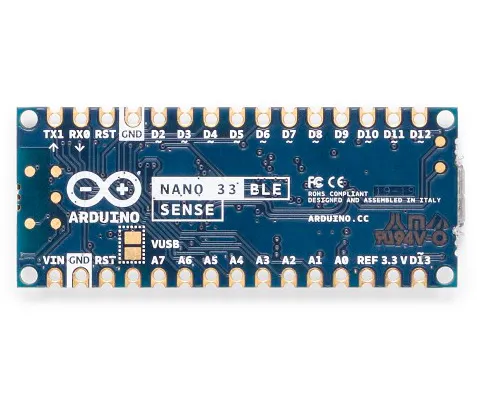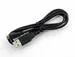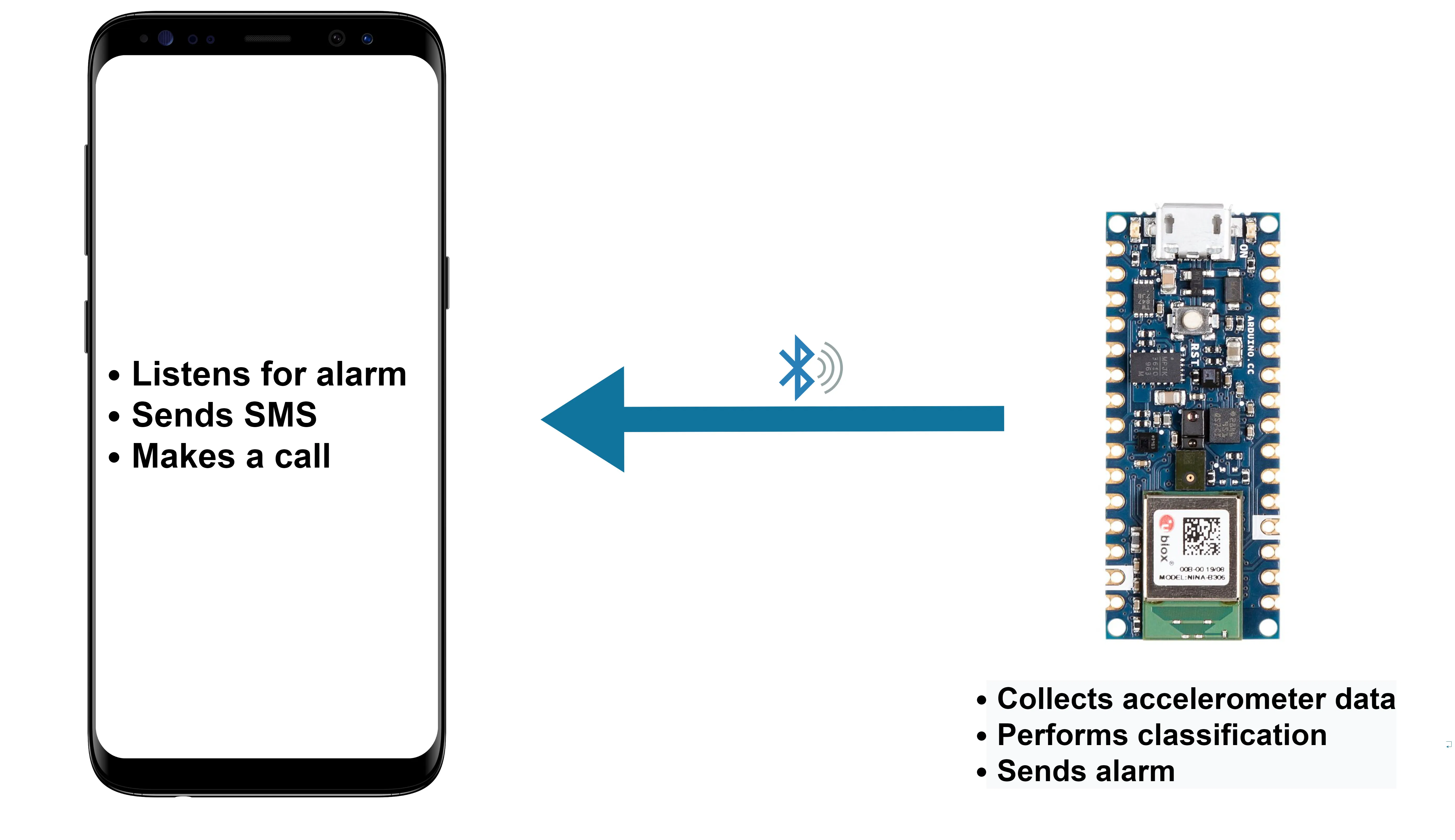Published

# Epilet - bracelet for epilepsy attacks

Make sure you get the help you need, when you need it.

IntermediateWork in progress3,986

## Things used in this project

### Hardware componentsArduino Nano 33 BLE Sense
×1USB-A to Micro-USB Cable
×1

### Software apps and online servicesArduino IDEEdge Impulse StudioMIT App Inventor

## Schematics

### Scheme## Code

### INO file

C/C++
Use this ino file in arduino.
```#include <modulg-project-1_inference.h>
#include <Arduino_LSM9DS1.h>
#include <ArduinoBLE.h>

// BLE Battery Service
BLEService predictionService("382d6dfe-a1b3-11eb-bcbc-0242ac130002");

// BLE Battery Level Characteristic
BLEUnsignedCharCharacteristic predictionChar("382d6dff-a1b3-11eb-bcbc-0242ac130002",  // standard 16-bit characteristic UUID
BLERead | BLENotify); // remote clients will be able to get notifications if this characteristic changes

/* Constant defines -------------------------------------------------------- */
#define CONVERT_G_TO_MS2    9.80665f

/* Private variables ------------------------------------------------------- */
static bool debug_nn = false; // Set this to true to see e.g. features generated from the raw signal
static uint32_t run_inference_every_ms = 200;
static float buffer[EI_CLASSIFIER_DSP_INPUT_FRAME_SIZE] = { 0 };
static float inference_buffer[EI_CLASSIFIER_DSP_INPUT_FRAME_SIZE];
int counter = 0;
int indicator = 0;
float oldPrediction = 0;
long previousMillis = 0;
long previousUpdateMillis = 0;

void updatePrediction();
void run_inference_background();

void setup()
{
// put your setup code here, to run once:
Serial.begin(115200);
Serial.println("Edge Impulse Inferencing Demo");

//while (!Serial);

pinMode(LED_BUILTIN, OUTPUT); // initialize the built-in LED pin to indicate when a central is connected

// begin initialization
if (!BLE.begin()) {
Serial.println("starting BLE failed!");

while (1);
}

if (!IMU.begin()) {
ei_printf("Failed to initialize IMU!\r\n");
}
else {
ei_printf("IMU initialized\r\n");
}

if (EI_CLASSIFIER_RAW_SAMPLES_PER_FRAME != 3) {
ei_printf("ERR: EI_CLASSIFIER_RAW_SAMPLES_PER_FRAME should be equal to 3 (the 3 sensor axes)\n");
return;
}

BLE.setLocalName("Epilet");
predictionChar.writeValue(oldPrediction); // set initial value for this characteristic

Serial.println("Bluetooth device active, waiting for connections...");
}

void ei_printf(const char *format, ...) {
static char print_buf = { 0 };

va_list args;
va_start(args, format);
int r = vsnprintf(print_buf, sizeof(print_buf), format, args);
va_end(args);

if (r > 0) {
Serial.write(print_buf);
}
}

void run_inference_background()
{
// wait until we have a full buffer
delay((EI_CLASSIFIER_INTERVAL_MS * EI_CLASSIFIER_RAW_SAMPLE_COUNT) + 100);

// This is a structure that smoothens the output result
// With the default settings 70% of readings should be the same before classifying.
ei_classifier_smooth_t smooth;
ei_classifier_smooth_init(&smooth, 10 /* no. of readings */, 7 /* min. readings the same */, 0.8 /* min. confidence */, 0.3 /* max anomaly */);

while (1) {
// copy the buffer
memcpy(inference_buffer, buffer, EI_CLASSIFIER_DSP_INPUT_FRAME_SIZE * sizeof(float));

// Turn the raw buffer in a signal which we can the classify
signal_t signal;
int err = numpy::signal_from_buffer(inference_buffer, EI_CLASSIFIER_DSP_INPUT_FRAME_SIZE, &signal);
if (err != 0) {
ei_printf("Failed to create signal from buffer (%d)\n", err);
return;
}

// Run the classifier
ei_impulse_result_t result = { 0 };

err = run_classifier(&signal, &result, debug_nn);
if (err != EI_IMPULSE_OK) {
ei_printf("ERR: Failed to run classifier (%d)\n", err);
return;
}

// print the predictions
ei_printf("Predictions ");
ei_printf("(DSP: %d ms., Classification: %d ms., Anomaly: %d ms.)",
result.timing.dsp, result.timing.classification, result.timing.anomaly);
ei_printf(": ");

// ei_classifier_smooth_update yields the predicted label
const char *prediction = ei_classifier_smooth_update(&smooth, &result);

if (*prediction == '1') {
counter++;
if (counter == 20) {
//ime funkcije, ki bo odstevala
//tukaj printamo da je epilepsija
ei_printf("epilepsija\n");
indicator = 1;
counter = 0;
long currentMillis = millis();
if (currentMillis - previousMillis >= 200) {
previousMillis = currentMillis;
updatePrediction();
}
}

}
else {
counter = 0;
ei_printf("ni epilepsija\n");
indicator = 0;
long currentMillis = millis();
if (currentMillis - previousMillis >= 200) {
previousMillis = currentMillis;
updatePrediction();
}

}

ei_printf("%s ", prediction);
// print the cumulative results
ei_printf(" [ ");
for (size_t ix = 0; ix < smooth.count_size; ix++) {
ei_printf("%u", smooth.count[ix]);
if (ix != smooth.count_size + 1) {
ei_printf(", ");
}
else {
ei_printf(" ");
}
}
ei_printf("]\n");

delay(run_inference_every_ms);
}

ei_classifier_smooth_free(&smooth);
}

void loop()
{
// wait for a BLE central
BLEDevice central = BLE.central();
if (central) {
Serial.print("Connected to central: ");
// print the central's BT address:
// turn on the LED to indicate the connection:
digitalWrite(LED_BUILTIN, HIGH);

// check the battery level every 200ms
// while the central is connected:
while (central.connected()) {
long currentMillis = millis();
// if 200ms have passed, check the battery level:
if (currentMillis - previousMillis >= 200) {
previousMillis = currentMillis;
updatePrediction();
}

// Determine the next tick (and then sleep later)
uint64_t next_tick = micros() + (EI_CLASSIFIER_INTERVAL_MS * 1000);

// roll the buffer -3 points so we can overwrite the last one
numpy::roll(buffer, EI_CLASSIFIER_DSP_INPUT_FRAME_SIZE, -3);

// read to the end of the buffer
buffer[EI_CLASSIFIER_DSP_INPUT_FRAME_SIZE - 3],
buffer[EI_CLASSIFIER_DSP_INPUT_FRAME_SIZE - 2],
buffer[EI_CLASSIFIER_DSP_INPUT_FRAME_SIZE - 1]
);

buffer[EI_CLASSIFIER_DSP_INPUT_FRAME_SIZE - 3] *= CONVERT_G_TO_MS2;
buffer[EI_CLASSIFIER_DSP_INPUT_FRAME_SIZE - 2] *= CONVERT_G_TO_MS2;
buffer[EI_CLASSIFIER_DSP_INPUT_FRAME_SIZE - 1] *= CONVERT_G_TO_MS2;

// and wait for next tick
uint64_t time_to_wait = next_tick - micros();
delay((int)floor((float)time_to_wait / 1000.0f));
delayMicroseconds(time_to_wait % 1000);
}
// when the central disconnects, turn off the LED:
digitalWrite(LED_BUILTIN, LOW);
Serial.print("Disconnected from central: ");
}

}

void updatePrediction() {
float prediction = indicator;
long currentMillis = millis();

if (prediction != oldPrediction || (currentMillis - previousUpdateMillis) >= 5000) {      // if the battery level has changed
previousUpdateMillis = currentMillis;
predictionChar.writeValue(prediction);  // and update the battery level characteristic
oldPrediction = prediction;           // save the level for next comparison
}
}

#if !defined(EI_CLASSIFIER_SENSOR) || EI_CLASSIFIER_SENSOR != EI_CLASSIFIER_SENSOR_ACCELEROMETER
#error "Invalid model for current sensor"
#endif
```

### Github repository

All code including libraries. Add library to arduino. You can compress it as .zip and include as zip library. Sketch --> Include library --> Add .ZIP Library...

## Credits

### Monika Bogataj

1 project • 6 followers

### Aleksander hrastič

1 project • 5 followers

### Jaka Jemec

1 project • 5 followers

### Timon Kalagasidis

1 project • 5 followers